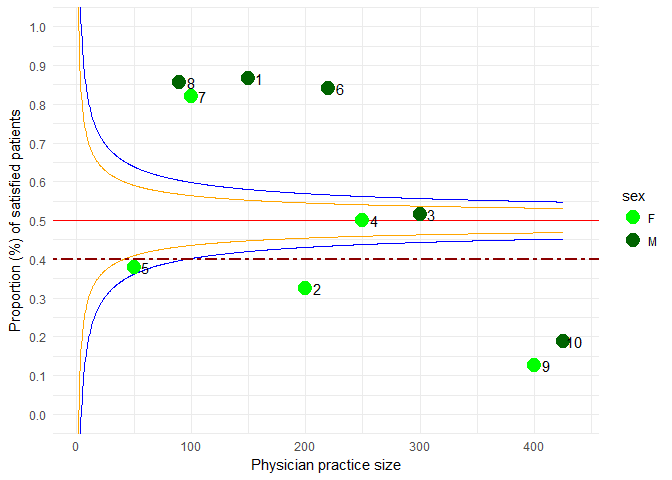Funnel Plots for Proportion Data

Overview

The funnelR package provides a flexible framework for creating funnel plots for proportion data. A funnel plot is a powerful visualization in the analysis of unit level performance relative to some criterion. It readily allows identification of units that are In Control or Extreme according to a benchmark at specified level of confidence (e.g.95%).

Framed this way, a funnel plot can be applied to any number of fields of study to monitor and identify units that deviate from what is considered typical. For example, it could be used to differentiate schools that are high, average or low performing on a standardized test according to a National or State benchmark. From a quality improvement point of view, they might help identify which hospitals have extreme mortality or surgical complication rates relative to a benchmark prescribed by a government body.

The funnelR package provides many options to specify elements of a funnel plot including user defined: control limits, benchmarks, and estimation methods. It also has the capability to write scored results (i.e. a variable that records whether a unit is In Control or Extreme according to the specifications of the funnel plot) to your sample data set. This variable might then be included in further analysis such as cross-tabulations (e.g. stratification) or regression modeling (e.g. covariate).

While many flavors of funnel plots exist (rates, ratios, etc.), the current package considers funnel plots assuming proportion data that is binomially distributed. The interested reader is referred to Spiegelhalter (2005) for further details.

Data for Examples

To use the funnelR package, your sample data must follow some basic conventions:

1. One observation per row.
2. The numerator variable must be named n.
3. The denominator variable must be named d.

The following sample data set will be used for illustrating the features of the package.

1. id: Physician ID.
2. sex: Physician Sex.
3. n: Number of patients who rated their recent care as satisfactory.
4. d: Total number of patients under the care of the physician.
my_data <- data.frame(id=c(1,2,3,4,5,6,7,8,9,10),
sex=c('M','F','M','F','F','M','F','M','F','M'),
n=c(130,65,155,125,19,185,82,77,50,80),
d=c(150,200,300,250,50,220,100,90,400,425)
)
knitr::kable(my_data)
id sex n d
1 M 130 150
2 F 65 200
3 M 155 300
4 F 125 250
5 F 19 50
6 M 185 220
7 F 82 100
8 M 77 90
9 F 50 400
10 M 80 425

Example 1

Let’s model the sample data using a funnel plot. This analysis might help shed some insight on which physicians are receiving satisfactory ratings.

Consider a factitious benchmark of 50% being considered the norm. We can draw a funnel plot with 80% and 95% confidence limits and see who falls where. For this example we will use the exact method. Note the step must be an integer for the exact method.

library(funnelR)
my_limits <- fundata(input=my_data,
benchmark=0.50,
alpha=0.80,
alpha2=0.95,
method='exact',
step=1)
my_plot <- funplot(input=my_data,
fundata=my_limits)
my_plot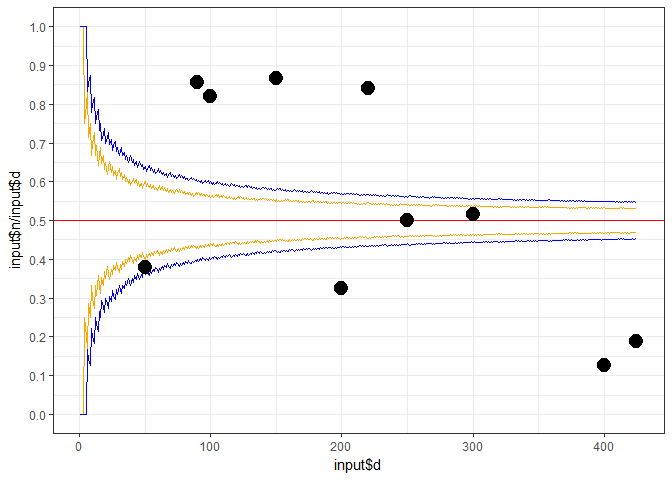Example 2

Let’s repeat Example 1, but set the method to approximate. We will need to set the step parameter to something reasonably small to produce the two sets of smooth confidence limits.

my_limits2 <- fundata(input=my_data,
benchmark=0.50,
alpha=0.80,
alpha2=0.95,
method='approximate',
step=0.5)
my_plot2 <- funplot(input=my_data,
fundata=my_limits2)
my_plot2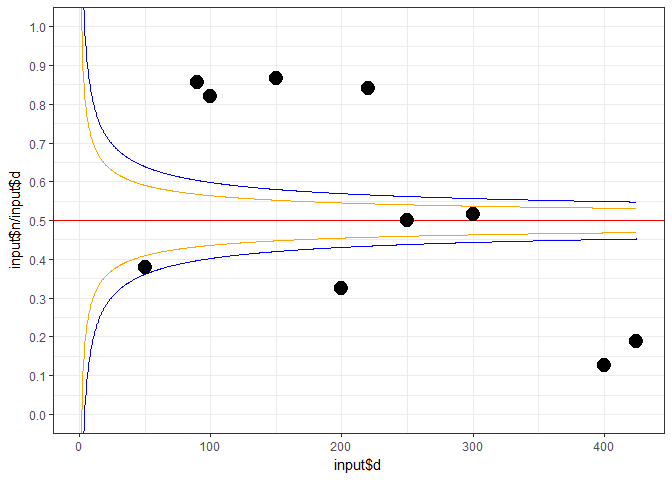Example 3

As previously mentioned, the funnelR package is capable of scoring your sample data. Scoring here refers to returning a variable in your sample data which records whether each observation is In Control or Extreme according to the specifications of the funnel plot. This can be useful in further analyses of your data (e.g. a stratification variable).

We’ll score the sample data according to the specifications in Example 2.

my_score <- funscore(input=my_data,
benchmark=0.50,
alpha=0.80,
alpha2=0.95,
method='approximate')
knitr::kable(my_score)
id sex n d r z score score2
1 M 130 150 0.8666667 8.9814624 Extreme Extreme
2 F 65 200 0.3250000 4.9497475 Extreme Extreme
3 M 155 300 0.5166667 0.5773503 In Control In Control
4 F 125 250 0.5000000 0.0000000 In Control In Control
5 F 19 50 0.3800000 1.6970563 Extreme In Control
6 M 185 220 0.8409091 10.1129979 Extreme Extreme
7 F 82 100 0.8200000 6.4000000 Extreme Extreme
8 M 77 90 0.8555556 6.7461923 Extreme Extreme
9 F 50 400 0.1250000 15.0000000 Extreme Extreme
10 M 80 425 0.1882353 12.8543881 Extreme Extreme

The variable score and score2 correspond to the parameters alpha and alpha2, respectively.

We can take the analysis one step further and produce a funnel plot, which is colored by score2 pretty painlessly!

my_plot3 <- funplot(input=my_score,
fundata=my_limits2,
byvar="score2")
my_plot3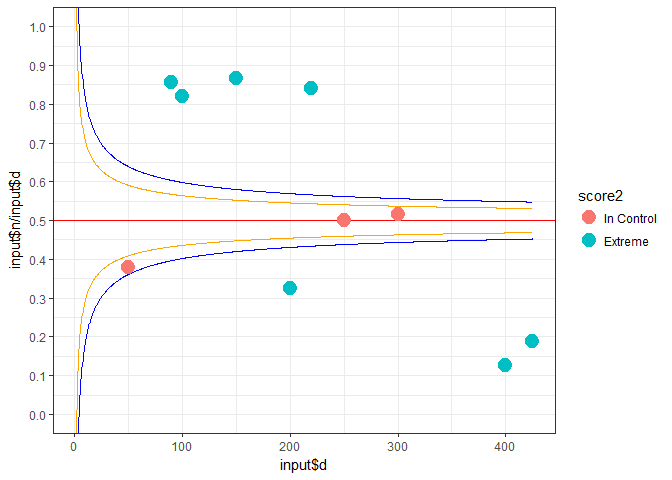Finally, since sex is also present on the sample data set, we can also color the funnel plot by this too!

my_plot4 <- funplot(input=my_score,
fundata=my_limits2,
byvar="sex")
my_plot4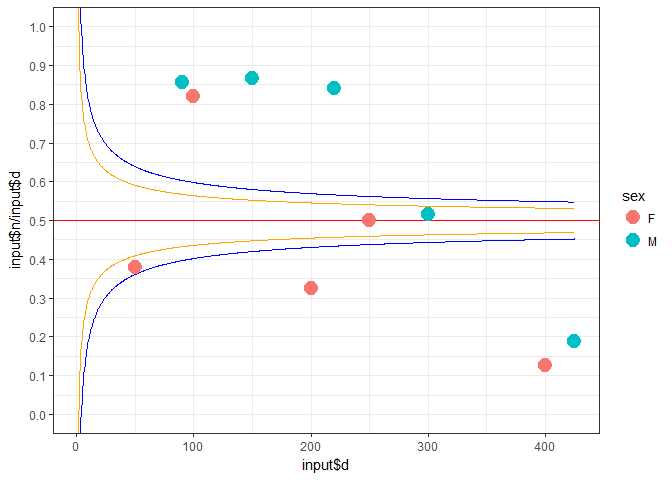Example 4

The funplot function is essentially a wrapper for ggplot2, which will return a base funnel plot as a ggplot object. You can leverage your existing ggplot2 knowledge to customize the funnel plot.

We will use produce a customized funnel plot using the specifications from Example 2. This time, we will add the following features:

1. Custom axes text.
2. Add a secondary benchmark as a reference.
3. Change the plot theme.
4. Change the colors of the points.
5. Label each point by the id variable.
library(ggplot2)
#> Warning: package 'ggplot2' was built under R version 3.4.3
my_plot4_mod <- my_plot4 +
labs(x="Physician practice size", y="Proportion (%) of satisfied patients") +
geom_hline(yintercept=0.40, colour="darkred", linetype=6, size=1) +
theme_minimal() +
scale_colour_manual(values=c("green","darkgreen")) +
geom_text(aes(label=id), colour="black", size=4, nudge_x=10)
my_plot4_mod